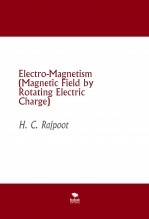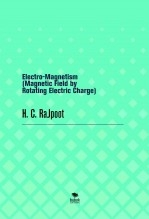This website, whose manager is Bubok Publishing, s.l., uses cookies (small data files that get stored in your browser), your own data and third parties' data as well, for the operation of the site (necessary), analytics (anonymous analysis of your activity on the website) and social media activity (so that you can interact through them). You can read our política de cookies. You can accept, reject, manage or view more information about said cookies by clicking on the corresponding button.

# HARISH CHANDRA RAJPOOT

He is studying for a Ph.D. at Indian Institute of Technology Bombay. He did M. Tech in Production Engineering from Indian Institute of Technology Delhi. He did B.Tech. (Hons) in Mechanical Engineering from Madan Mohan Malaviya University of Technology (Formerly M.M.M. Engg. College), Gorakhpur, U.P. India. He completed his High School at D.A.V. Inter College, Mahoba (UP) & Intermediate from Oxford Model Inter College, Syam Nagar, Kanpur, U.P. India. He had been guided & inspired by his respected Guru Mr. Upendra Sir, a well-renowned & experienced teacher of Physics, for research work.

He made independent research on 'Solid Angle' & applied it in Radiometry/Photometry in Fundamental Physics. He applied it to the point sources of radiation emitting energy uniformly in all directions. He modified Lambert's Cosine Formula to apply for higher accuracy & to analytically compute Illuminance. He proposed the 'Approximation Theorem of Solid Angle' for symmetrical 2-D figures. His Approximation-formula gives the results very closer to the values calculated by the analytic method.

He proposed the 'Theory of Polygon' which is applicable to all polygons to analytically & graphically compute the solid angle subtended at any arbitrary point in the space. He consequently, applying his theory of polygon derived a generalized formula for all five regular polyhedra & analyzed all 13 Archimedean solids to compute all the important parameters or dimensions i.e. inner radius, outer radius, mean radius, surface area & volume.

He derived & proved HCR's Theorem to analytically compute the V-cut angle for folding two co-planar, meeting at angle bisector, about their intersecting straight edges so as to coincide their new edges. This theorem is very useful for making Pyramidal Flat Containers with Regular Polygonal Bases, Right Pyramids & Polyhedrons with polygonal & trapezoidal faces using a sheet of paper, polymer, metal, or alloy which can be easily bent and butt-joined at the mating edges. He, using his theorem, also derived HCR's Corollary to analytically compute the dihedral angle between two folded planes.

He derived a unique formula for permutations of alphabetic words, positive integral numbers & all other linear permutations to calculate the position (hierarchical rank) of any linear permutation in the ordered sequence. It was certified by the International Journal of Mathematics & Physical Sciences Research in March 2014. Consequently, he, using HCR's Rank Formula-1 proved that the factorial of any number can be expanded as a sum of finite terms. This series was named as HCR's Series certified by the International Organization of Scientific Research. He applied his formula-1 for computing the rank of circular permutations He further gave HCR's Rank Formula-2 for computing the rank of any linear permutations when repetition of articles is permitted.

He generalized

1. formula for regular polyhedra (all five platonic solids)

2. formula for an infinite class of uniform polyhedra with regular n-gonal & trapezoidal faces

3. formula for an infinite class of trapezohedrons (uniform polyhedrons with congruent right kite faces)

4. a formula for all regular spherical polygons & derived formula to compute the radii of circles internally as well as externally touching three external tangent circles.

5. a formula to compute the distance between any two points on the globe.

Mr. H. C. Rajpoot wrote his first book Advanced Geometry based on his research articles in Applied Mathematics & Radiometry for higher education which were first published by Notion Press, Chennai, India in April 2014. This book is based on research in higher education to put advanced articles into the practice of education.

He was given Best Paper Award for his outstanding performance in his research paper 'Magnetic Field Generated by Rotating Electric Charge' in Young Scholar's National Research Writing Competition organized by Mind Share Yuva on 8th March 2021.

He is an active reviewer for the Journal of Pure and Applied Mathematics (IF: 4.0), Pulsus Group since Feb 2023.

### Electro-Magnetism (Magnetic Field by Rotating Electric [...]The author Mr H. C. Rajpoot (Master of Technology at I.I.T. Delhi) has derived numerous mathematical formula used to analytically compute the [...] See book

### Electro-Magnetism (Magnetic Field by Rotating Electric [...]This book mainly deals with the new articles based on research work of the author in Electro-Magnetism. The research articles in this book are [...] See book